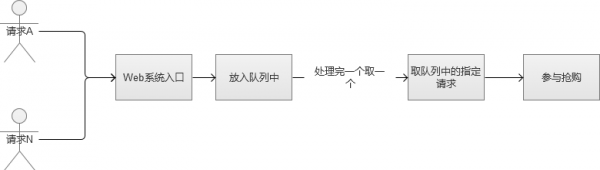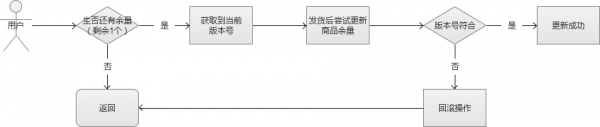# php如何应对秒杀抢购高并发思路 （乐观锁，悲观锁）

http://www.cnblogs.com/weishanyun/p/6891994.html?utm_source=tuicool&utm_medium=referral

20*500/0.25 = 40000 （4万QPS）

3. 重启与过载保护

1. 超发的原因

 1 2 3 4 5 6 7 8 9 10 11 12 13 14 15 16 17 18 19 20 21 22 23 24 25 26 27 28 29 30 31 32 33 34 35 36 37 38 39 40 41 42 43 44 45 46 47 48 fetch_assoc();   if($row['number']>0){//高并发下会导致超卖  if($row['number']<$number){  return insertLog('库存不够',3,$username);       }       $order_sn=build_order_no();  //库存减少  $sql="update ih_store set number=number-{$number} where sku_id='$sku_id' and number>0";       $store_rs=mysqli_query($conn,$sql);  if($store_rs){           //生成订单           insertOrder($order_sn,$user_id,$goods_id,$sku_id,$price,$username,$number);  insertLog('库存减少成功',1,$username);       }else{           insertLog('库存减少失败',2,$username);  }  }else{  insertLog('库存不够',3,$username);   }     ?>1 2 3 4 5 6 7 8 9 10 11 12 13 14 15 16 17 18 19 20 21 22 23 24 25 26 27 28 29 30 31 32 33 34 35 36 37 38 39 40 41 42 43 44 fetch_assoc(); if($row['number']>0){  //生成订单  $order_sn=build_order_no();     $sql="insert into ih_order(order_sn,user_id,goods_id,sku_id,price)  values('$order_sn','$user_id','$goods_id','$sku_id','$price')";     $order_rs=mysqli_query($conn,$sql);  //库存减少  $sql="update ih_store set number=number-{$number} where sku_id='$sku_id'";     $store_rs=mysqli_query($conn,$sql);  if($store_rs){       echo '库存减少成功';         insertLog('库存减少成功');         mysqli_query($conn,"COMMIT");//事务提交即解锁  }else{  echo '库存减少失败';  insertLog('库存减少失败');  } }else{  echo '库存不够';  insertLog('库存不够');  mysqli_query($conn,"ROLLBACK"); } ?>

3. FIFO队列思路4. 文件锁的思路

 1 2 3 4 5 6 7 8 9 10 11 12 13 14 15 16 17 18 19 20 21 22 23 24 25 26 27 28 29 30 31 32 33 34 35 36 37 38 39 40 41 42 43 44 45 46 47 48 49 50 fetch_assoc(); if($row['number']>0){//库存是否大于0  //模拟下单操作  $order_sn=build_order_no();     $sql="insert into ih_order(order_sn,user_id,goods_id,sku_id,price)  values('$order_sn','$user_id','$goods_id','$sku_id','$price')";     $order_rs = mysqli_query($conn,$sql);  //库存减少  $sql="update ih_store set number=number-{$number} where sku_id='$sku_id'";     $store_rs = mysqli_query($conn,$sql);  if($store_rs){       echo '库存减少成功';         insertLog('库存减少成功');         flock($fp,LOCK_UN);//释放锁  }else{  echo '库存减少失败';  insertLog('库存减少失败');  } }else{  echo '库存不够';  insertLog('库存不够'); } fclose($fp);      ?>

5. 乐观锁思路1 2 3 4 5 6 7 8 9 10 11 12 13 14 15 16 17 18 19 20 21 22 23 24 25 26 27 28 29 30 31 32 33 34 35 36 37 38 39 40 41 42 43 44 45 46 47 48 49 50 51 52 53 54 55 56 57 connect('127.0.0.1', 6379);  echo $mywatchkey = $redis->get("mywatchkey");   /*   //插入抢购数据  if($mywatchkey>0)  {  $redis->watch("mywatchkey");   //启动一个新的事务。     $redis->multi();  $redis->set("mywatchkey",$mywatchkey-1);  $result = $redis->exec();  if($result) {       $redis->hSet("watchkeylist","user_".mt_rand(1,99999),time());  $watchkeylist = $redis->hGetAll("watchkeylist");  echo "抢购成功！ ";  $re = $mywatchkey - 1;   echo "剩余数量：".$re."
";         echo "用户列表：
";  			        print_r($watchkeylist);  }else{  echo "手气不好，再抢购！";exit;  }   }else{  //$redis->hSet("watchkeylist","user_".mt_rand(1,99999),"12");  			     //  $watchkeylist =$redis->hGetAll("watchkeylist");  			        echo "fail！
";            echo ".no result
";         echo "用户列表：
";  			      //  var_dump($watchkeylist);   }*/ $rob_total = 100;   //抢购数量  			if($mywatchkey<=$rob_total){  			    $redis->watch("mywatchkey");  $redis->multi(); //在当前连接上启动一个新的事务。  			    //插入抢购数据  			    $redis->set("mywatchkey",$mywatchkey+1);  			    $rob_result = $redis->exec();  			    if($rob_result){  $redis->hSet("watchkeylist","user_".mt_rand(1, 9999),$mywatchkey);  $mywatchlist = $redis->hGetAll("watchkeylist");  echo "抢购成功！ ";    echo "剩余数量：".($rob_total-$mywatchkey-1)." ";  echo "用户列表： ";  var_dump($mywatchlist);  			    }else{  			          \$redis->hSet("watchkeylist","user_".mt_rand(1, 9999),'meiqiangdao');  			        echo "手气不好，再抢购！";exit;  			    }  			}  			?>

暂时只想到这些，如果有什么新方法可以评论区讨论交流